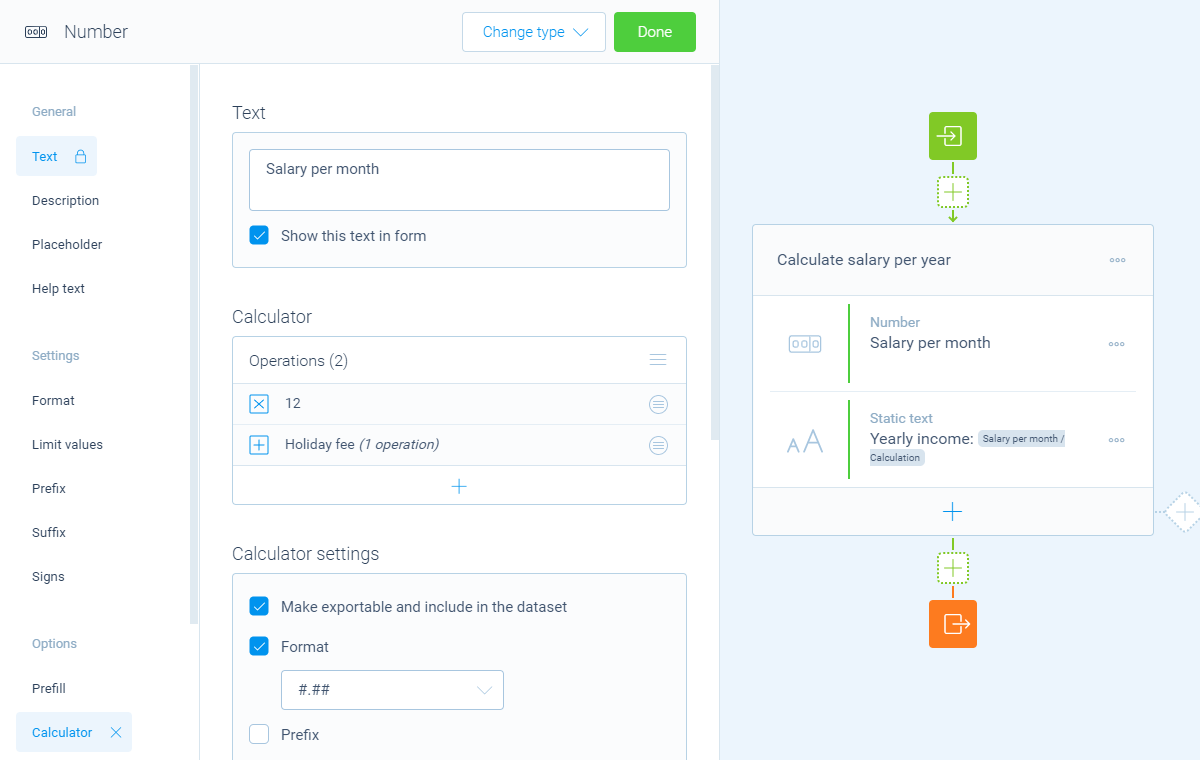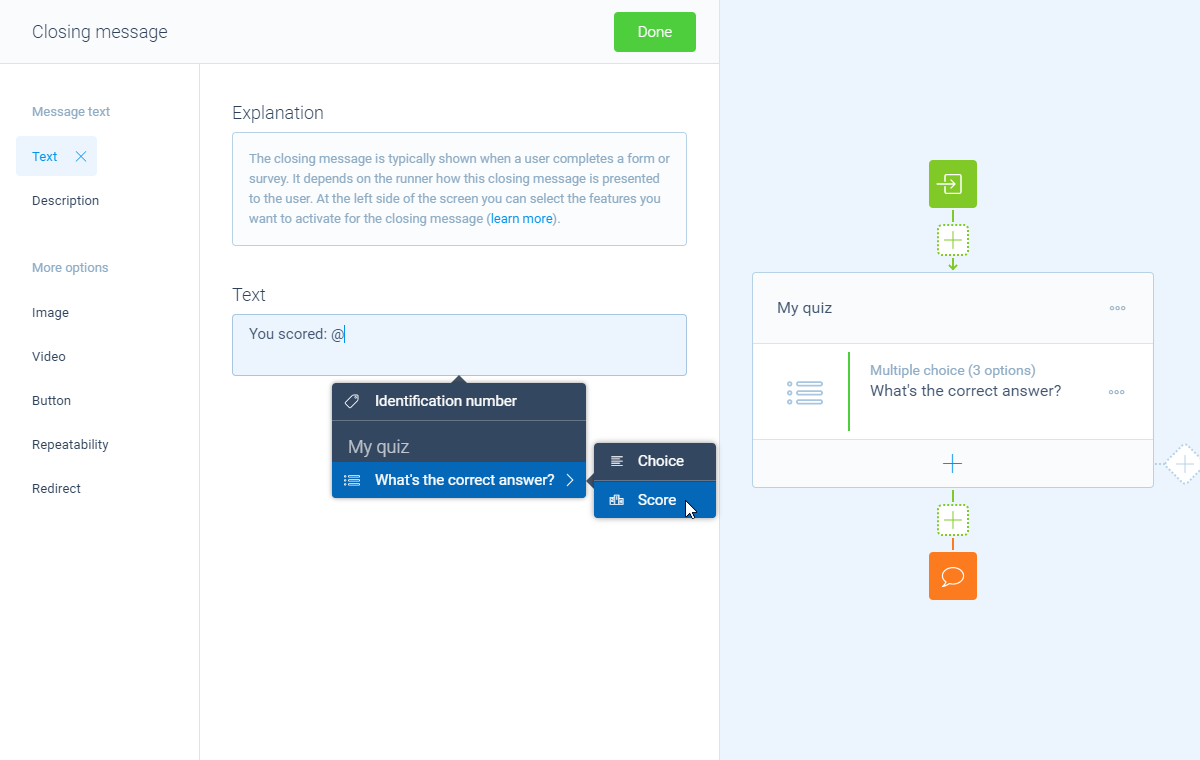Using logic4 Min.2 Min.

# How to add instant scores and calculations inside question blocks

For lots of question blocks we made it easier to use the most common calculator features, without adding a separate calculator block. For example to quickly add scores to your quiz questions.

## When to use

The calculator block is a separate action block in your form. But sometimes you just want to perform a relatively easy calculation, like adding a score based on the given answer(s) to a multiple choice questions.

Such quick calculations can also be done without the need of a separate calculation block, by using instant scores or instant calculations inside question blocks. For example:

• Instantly score the answer options of a multiple choice question in a quiz;
• Instantly perform an advanced formula inside a number block and use the entered number as input for the formula.

## How to use

In the article you're reading now we describe how to perform calculations directly inside question blocks. This helps you to easily perform calculations, without the need for one or more loose calculator blocks in your form. If you need more advanced calculation actions, please have a look at this article about the calculator block.

### Enable instant scoring

For all question blocks that have answer options in them, you can instantly score those answer options to get a score out of that block. By enabling the `Score` feature inside a question block you can enter the desired scores for the various answer options right away, without adding a separate calculator block.

This question block will now save the selected option(s) by the respondent, but will also save the score outcome based on the selected option(s).

We have a separate article that describes more about using scores in the calculator. How to use scores in your calculations.

### Enable instant calculations

For blocks that output a number, it is possible to instantly use the full calculator features, without adding a separate calculator block. By enabling the `Calculator` feature inside a question block you get access to the full calculator.

The calculator then uses the entered value of the pertaining question as the initial value and you can instantly perform the right calculations with that value.

This question block will now save the entered number input by the respondent, but will also save the calculator outcome.Example of an instant calculation to calculate the yearly salary based on the entered monthly salary.

We have a separate article about the capabilities of the calculator block. How to use the calculator block.

### Use outcome

The outcome of the integrated scoring/calculator in each question block is also directly available. You can use that for all kinds of actions, as also described in the article about how to use calculator outcomes:

• Save the outcome in dataset;
• Show the outcome to your respondents in your form (piping logic);
• Use the outcome as input for an other calculator block1;
• Use the outcome as logic condition to determine the follow-up (branch logic).Example of showing the outcome of a score in the closing message.

#### Ad 1: Scores in other calculator block

If you have added scores inside question blocks, you can use those scores in other calculators instantly. In that case the value that's entered in the question block is the place where you manage the score values.

It's also possible to use new scoring values inside a new calculator block. Those can differ from the scores that are entered in the question block itself. Those new score values are only valid within the pertaining calculator block.

The calculator block has lots of features, so we have several ways to learn all about it.

### Help center

Our help articles help you out on all different aspects of the calculator:

### Overviews

We also made some overviews of the capabilities that the calculator block provides: